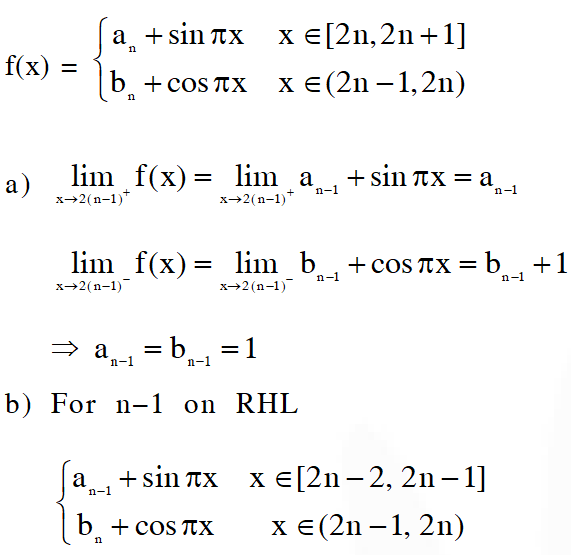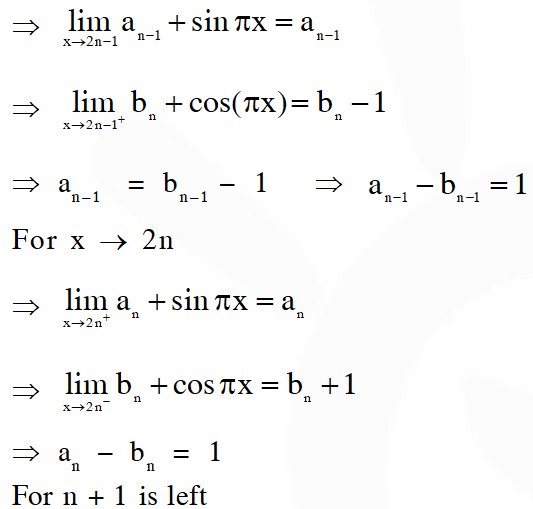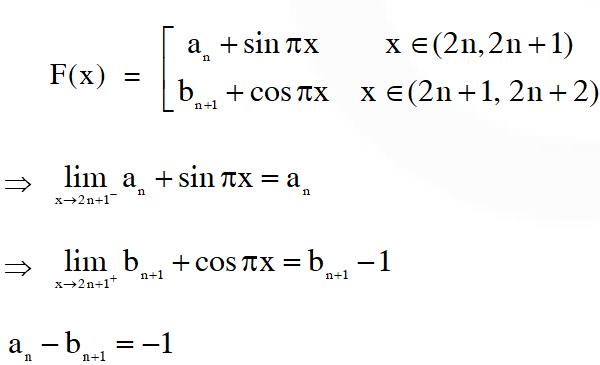Download the eSaral app and start learning from Kota's top IITians and doctors.

# Continuity - JEE Advanced Previous Year Questions with Solutions

JEE Advanced Previous Year Questions of Math with Solutions are available at eSaral. Practicing JEE Advanced Previous Year Papers Questions of mathematics will help the JEE aspirants in realizing the question pattern as well as help in analyzing weak & strong areas. eSaral helps the students in clearing and understanding each topic in a better way. eSaral also provides complete chapter-wise notes of Class 11th and 12th both for all subjects. Besides this, eSaral also offers NCERT Solutions, Previous year questions for JEE Main and Advance, Practice questions, Test Series for JEE Main, JEE Advanced and NEET, Important questions of Physics, Chemistry, Math, and Biology and many more. Download eSaral app for free study material and video tutorials.
Q. For every integer $n$, let $a_{n}$ and $b_{n}$ be real numbers. Let function $f: \mathbb{R} \rightarrow \mathbb{R}$ be given by $f(\mathrm{x})=\left\{\begin{array}{ll}{\mathrm{a}_{\mathrm{n}}+\sin \pi \mathrm{x},} & {\text { for } \quad \mathrm{x} \in[2 \mathrm{n}, 2 \mathrm{n}+1]} \\ {\mathrm{b}_{\mathrm{n}}+\cos \pi \mathrm{x},} & {\text { for } \quad \mathrm{x} \in(2 \mathrm{n}-1,2 \mathrm{n})}\end{array}, \text { for all integers } \mathrm{n}\right.$ If ƒ is continuous, then which of the following holds(s) for all n ? (A) $a_{n-1}-b_{n-1}=0$ (B) $a_{n}-b_{n}=1$ (C) $a_{n}-b_{n+1}=1$ (D) $a_{n-1}-b_{n}=-1$ [JEE 2012, 4M]
Ans. (B,D)Q. For every pair of continuous function $f, g:[0,1] \rightarrow$ such that $\max \{f(x): x \in[0,1]\}=$ $\max \{g(x): x \in[0,1]\},$ the correct statement(s) is(are) : (A) $(f(\mathrm{c}))^{2}+3 f(\mathrm{c})=(\mathrm{g}(\mathrm{c}))^{2}+3 \mathrm{g}(\mathrm{c})$ for some $\mathrm{c} \in[0,1]$ (B) $(f(\mathrm{c}))^{2}+f(\mathrm{c})=(\mathrm{g}(\mathrm{c}))^{2}+3 \mathrm{g}(\mathrm{c})$ for some $\mathrm{c} \in[0,1]$ (C) $(f(\mathrm{c}))^{2}+3 f(\mathrm{c})=(\mathrm{g}(\mathrm{c}))^{2}+\mathrm{g}(\mathrm{c})$ for some $\mathrm{c} \in[0,1]$ (D) $(f(\mathrm{c}))^{2}=(\mathrm{g}(\mathrm{c}))^{2}$ for some $\mathrm{c} \in[0,1]$ [JEE(Advanced)-2014, 3]
Ans. (A,D) $f, \mathrm{g}[0,1] \rightarrow \mathrm{R}$ we take two cases. Let $f \& \mathrm{g}$ attain their common maximum value at $\mathrm{P}$. $\Rightarrow f(\mathrm{p})=\mathrm{g}(\mathrm{p})$ where $\mathrm{p} \in[0,1]$ let $f \& \mathrm{g}$ attain their common maximum value at different points. $\Rightarrow f(\mathrm{a})=\mathrm{M} \& \mathrm{g}(\mathrm{b})=\mathrm{M}$ $\Rightarrow f(\mathrm{a})-\mathrm{g}(\mathrm{a})>0 \& f(\mathrm{b})-\mathrm{g}(\mathrm{b})<0$ $\Rightarrow f(\mathrm{c})-\mathrm{g}(\mathrm{c})=0$ for some $\mathrm{c} \in[0,1]$ as $\mathrm{f}^{\prime} \& \quad$ g' are continuous functions $\Rightarrow f(\mathrm{c})-\mathrm{g}(\mathrm{c})=0$ for some $\mathrm{c} \in[0,1]$ for all cases. $\ldots(1)$ Option $(\mathrm{A}) \Rightarrow f^{2}(\mathrm{c})-\mathrm{g}^{2}(\mathrm{c})+3(f(\mathrm{c})-\mathrm{g}(\mathrm{c}))=0$ which is true from ( 1) Option (D) $\Rightarrow f^{2}(\mathrm{c})-\mathrm{g}^{2}(\mathrm{c})=0$ which is true from ( 1) Now, if we take $f(\mathrm{x})=1 \& \mathrm{g}(\mathrm{x})=1 \forall \mathrm{x} \in[0,1]$ options $(\mathrm{B}) \&(\mathrm{C})$ does not hold. Hence $\quad$ option $(\mathrm{A}) \&(\mathrm{D})$ are correct.
Q. Let $[\mathrm{x}]$ be the greatest integer less than or equal to $\mathrm{x}$. Then, at which of the following point(s) the function $f(\mathrm{x})=\mathrm{x} \cos (\pi(\mathrm{x}+[\mathrm{x}]))$ is discontinuous?b (A) x = –1 (B) x = 0 (C) x = 2 (D) x = 1 [JEE(Advanced)-2017, 4]
Ans. (A,C,D) $f(\mathrm{x})=\mathrm{x} \cos (\pi \mathrm{x}+[\mathrm{x}] \pi)$ $\Rightarrow f(\mathrm{x})=(-1)^{[\mathrm{x}]} \mathrm{x} \cos \pi \mathrm{x}$ Discontinuous at all integers except zero.